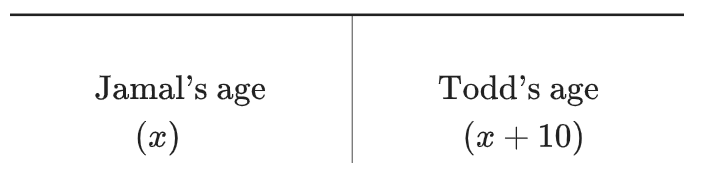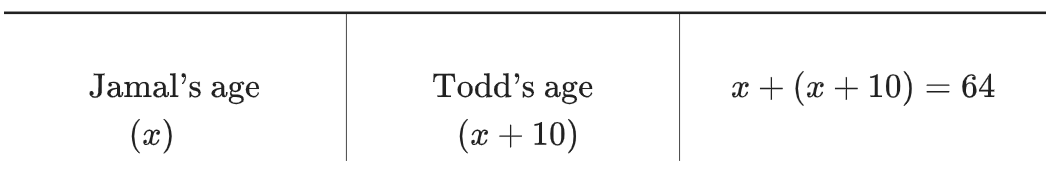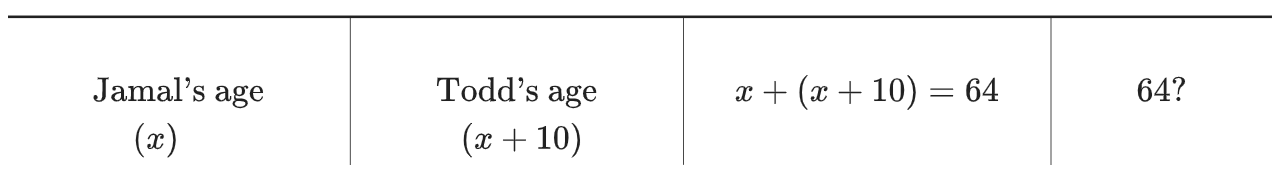### Home > CC3MN > Chapter 1 > Lesson 1.2.2 > Problem1-60

1-60.

Define a variable and then write and solve an equation for the problem below. Remember that you can use the $5$-D Process (described in the Lesson 1.1.3 Math Notes box) to help you get started. State your solution in a sentence.

Todd is $10$ years older than Jamal. The sum of their ages is $64$. How old are Todd and Jamal?

Rewrite the word problem as an equation or system of equations.

Have Jamal's age be $x$. Write Todd's age in terms of $x$.

1 row of 2 columns, labeled from left to right, as follows: Jamal's age, x, Todd's age, x + 10.

Third column added, labeled, x + (x+10) = 64.